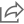Texas Instruments Scientific Calculator, TI36XPRO, W/Multi View, 3-1/3" X 7-1/4" X 3/4", Black
This product ad may change over timeShare

# Texas Instruments Scientific Calculator, TI36XPRO, W/Multi View, 3-1/3" X 7-1/4" X 3/4", Black

Advanced scientific calculator features a MultiView display and MathPrint capability. Enhanced math functionality makes this calculator ideal for computer science and engineering courses in which graphing technology may not be permitted. Easily input data, scroll through e…
Advanced scientific calculator features a MultiView display and MathPrint capability. Enhanced math functionality makes this calculator ideal for computer science and engineering courses in which graphing technology may not be permitted. Easily input data, scroll through entries, make edits and investigate patterns as you would on a graphing calculator. The MultiView display shows multiple calculations at the same time on screen. MathPrint shows math expressions, symbols and stacked fractions as they appear in textbooks. Convert fractions, decimals and terms, including Pi, into alternate representations. Key features include mode menu for easy, intuitive access to commands, memory variables to store real numbers and expressions, data list editor, function table, vectors and matrices, constants and conversions, and solvers (numeric equation, polynomial and system of linear equations). Dual-power scientific calculator runs on solar and battery power. 9.70 L. 6.60 W. 1.40 H.
See more
\$37.95
Visit Global Industrial

## Description

Advanced scientific calculator features a MultiView display and MathPrint capability. Enhanced math functionality makes this calculator ideal for computer science and engineering courses in which graphing technology may not be permitted. Easily input data, scroll through entries, make edits and investigate patterns as you would on a graphing calculator. The MultiView display shows multiple calculations at the same time on screen. MathPrint shows math expressions, symbols and stacked fractions as they appear in textbooks. Convert fractions, decimals and terms, including Pi, into alternate representations. Key features include mode menu for easy, intuitive access to commands, memory variables to store real numbers and expressions, data list editor, function table, vectors and matrices, constants and conversions, and solvers (numeric equation, polynomial and system of linear equations). Dual-power scientific calculator runs on solar and battery power. 9.70 L. 6.60 W. 1.40 H.